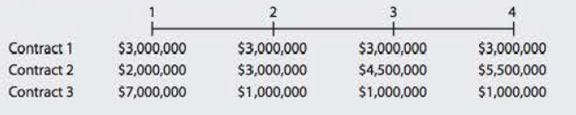Chapter 5, Problem 20PFundamentals of Financial Manageme...

15th Edition
Eugene F. Brigham + 1 other
ISBN: 9781337395250

Solutions

Chapter
SectionFundamentals of Financial Manageme...

15th Edition
Eugene F. Brigham + 1 other
ISBN: 9781337395250
Textbook Problem

PV OF A CASH FLOW STREAM A rookie quarterback is negotiating his first NFL contract. His opportunity cost is 7%. He has been offered three possible 4-year contracts. Payments are guaranteed, and they would be made at the end of each year. Terms of each contract are as follows:As his adviser, which contract would you recommend that he accept?

Summary Introduction

To calculate: The best stream for present value of cash flow

Introduction:

Future Value of Cash Flow:

If single cash flow put in an investment today which pays us compound interest how much does it grow over the period of time is known as future value of cash flow.

Explanation

Calculation of present value of cash flow stream at 7% compounding rate

 Contract 1 Contract 2 Contract 3 Year Cash in flow Present Value Cash in flow Present Value Cash in flow Present Value 1 3,000,000 2,803,738 2,000,000 1,869,159 7,000,000 6,542,056 2 3,000,000 2,620,316 3,000,000 2,620,316 1,000,000 873,439 3 3,000,000 2,448,894 4,500,000 3,673,340 1,000,000 816,298 4 3,000,000 2,288,686 5,500,000 4,195,924 1,000,000 762,895 Total PV 10,161,634 12,358,739 8,994,688

Table (1)

Working Note for present value:

Present value for year 1 and contract 1

Presentvaluefactor=CashFlow(1+Interestrate)Numberofyears=$3,000,000(1+0.07)1=$3,000,0001.07=$2,803,738.3 Present value for year 2 and contract 1 Presentvaluefactor=CashFlow(1+Interestrate)Numberofyears=$3,000,000(1+0.07)2=$2,620,316.1 Present value for year 3 and contract 1 Presentvaluefactor=CashFlow(1+Interestrate)Numberofyears=$3,000,000(1+0.07)3=$2,448,893.6 Present value for year 4 and contract 1 Presentvaluefactor=CashFlow(1+Interestrate)Numberofyears=$3,000,000(1+0.07)4=$2,288,685.6 Present value for year 1 and contract 2 Presentvaluefactor=CashFlow(1+Interestrate)Numberofyears=$2,000,000(1+0.07)1=$1,869,158.8 Present value for year 2 and contract 2 Presentvaluefactor=CashFlow(1+Interestrate)Numberofyears=$3,000,000(1+0

Still sussing out bartleby?

Check out a sample textbook solution.

See a sample solution

The Solution to Your Study Problems

Bartleby provides explanations to thousands of textbook problems written by our experts, many with advanced degrees!

Get Started

In what ways is economics a science?

Principles of Economics (MindTap Course List)

Explain the purpose of the work sheet.

College Accounting, Chapters 1-27

INFLATION AND INTEREST RATES In late 1980, the U.S. Commerce Department released new data showing inflation was...

Fundamentals of Financial Management, Concise Edition (with Thomson ONE - Business School Edition, 1 term (6 months) Printed Access Card) (MindTap Course List)